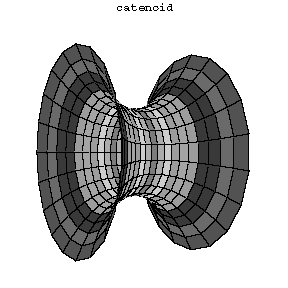# Catenoid

(diff) ← Older revision | Latest revision (diff) | Newer revision → (diff)

2010 Mathematics Subject Classification: Primary: 53A10 Secondary: 49Q05 [MSN][ZBL]

The surface formed by the revolution of a catenary $y=c\cosh x/c$ about the $x$-axis, where $c$ is a positive constant. The parametric equations for the catenoid are then $x = v \quad y = c \cosh \frac{v}{c} \sin u \quad z = c \cosh \frac{v}{c} \cos u\, .$ The catenoid is a minimal surface and it is the form realized by a soap film "stretched" over two wire discs the planes of which are perpendicular to the line joining their centres (see Fig. 1). The catenoid is a member of the one-parameter family of surfaces of revolution of the curves $y = a \cosh x/b$, which are sometimes also called catenoids. However only for the special choice of parameters $a = b$ the corresponding surface is a minimal surface and usually the word catenoid is used only for this particular case.

The catenoid is locally isometric to the helicoid and in fact such local isometry can be achieved as endpoint of a continuous one-parameter family of isometric deformations which are all minimal surfaces.Figure 1

How to Cite This Entry:
Catenoid. Encyclopedia of Mathematics. URL: http://encyclopediaofmath.org/index.php?title=Catenoid&oldid=38911
This article was adapted from an original article by BSE-3 (originator), which appeared in Encyclopedia of Mathematics - ISBN 1402006098. See original article